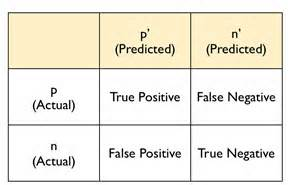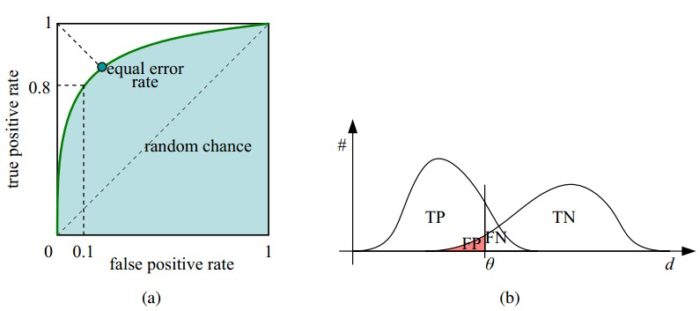# ROC曲线

### 1、混淆矩阵• 特指度(specificity)，即真负率(true negative rate) TNP = TN / (TN+FP), 是指被模型正确预测的负样本比例。
• 召回率(recall) r = TP / (TP + FN)，度量了被分类器正确预测的正样本比例。
• 精度(precision) p = TP / (TP + FP)，指在分类器断言为正类的样本中实际为正类的比例。

### 2、ROC曲线### 3、ROC代码

def roc1(scores):
# scores is predict
# scores is the target
m = len(scores)
pos_num = sum([i for i in scores])
neg_num = m - pos_num

fp, tp = [], []
FP, TP = 0, 0
# decent the scores
scores = sorted(scores, key=lambda x:x, reverse=True)
accs = []
for n,s in enumerate(scores):
TP = len([i for i in scores[0:n] if i == 1])
TN = len([i for i in scores[n:] if i == 0])
FP = len([i for i in scores[0:n] if i == 0])
accs.append([float(TP+TN)/m, s])
fp.append(float(FP) / neg_num)
tp.append(float(TP) / pos_num)

fp.append(1)
tp.append(1)
accs = sorted(accs, reverse=True)
return fp, tp, accs

def roc2(scores):
# scores is predict
# scores is the target
m = len(scores)
pos_num = sum([i for i in scores])
neg_num = m - pos_num

fp, tp = [], []
FP, TP = 0, 0
# decent the scores
scores = sorted(scores, key=lambda x:x, reverse=True)
threshold = 1.0
for s in scores:
if s < threshold:
fp.append(float(FP) / neg_num)
tp.append(float(TP) / pos_num)

if s == 1:
TP += 1
else:
FP += 1

fp.append(1)
tp.append(1)
return fp, tp

scores = [[1, 0.2], [1, 0.8], [1,0.89], [1, 0.98],
[0, 0.1], [0, 0.3], [0,0.34], [0, 0.56]]

fp1, tp1, accs1 = roc1(scores)
fp2, tp2 = roc2(scores)